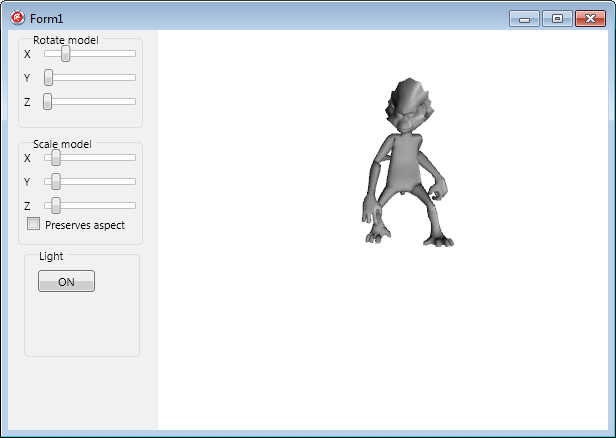# Applying Simple Customizations to an Imported 3D Model

This tutorial shows how to modify the scale, rotation angle, light, and position of an imported 3D model.

## Adjust the Imported 3D Model

After creating the form and having imported the wanted 3D model to the form (see Importing a 3D Model in a FireMonkey Application), follow the steps below to adjust the model.

### Use the Form Designer

You can adjust the position and rotation angles in the FireMonkey Form Designer by using the blue control cube attached to the model. The control cube appears when the model is selected in the Structure View:

• To move the model, perform a drag-and-drop operation with the control cube.
• To rotate the imported model, use the 3 blue handles of the control cube. Each handle rotates the model in the associated plane in space (that is, the x, y, or z vertex). When you click a handle, it becomes red to indicate that it is the active handle.

### Use the Object Inspector

You can scale, move, and rotate the model at design time by setting properties in the Object Inspector, as follows:

• Scale the imported model by setting each coordinate of the Scale property associated with each plane (X, Y, Z).
• Move the model by setting each coordinate of the Position property associated with each plane (X, Y, Z).
• Change the rotation angles by setting the RotationAngle properties of the model.

### Programmatically Adjust the 3D Model's Properties

1. If you are using a 3D Multi-Device Application, follow the steps in Creating a 2D Interface in a 3D Application to add 2D controls to the form.
2. Add the following 2D components to the form:
• TGroupBox with 3 TLabels and 3 TTrackBars to change the rotation angles. on each plan. Change the Text property to Rotate the model for the TGroupBox and to X, Y, and Z for the 3 labels.
• TGroupBox with 3 TLabels, 3 TTrackBars to change the scales of the model on each axis, and a TCheckBox to select whether the aspect is preserved when the model is scaled. Change the Text property to Scale the model for the TGroupBox, to X, Y, and Z for the 3 labels, and to Preserve aspect for the TCheckBox.
• TButton to set on or off the light on the scene. Change the Text property of the button to ON.
The form should look like this:3. In the Object Inspector, set the following :
4. Double-click each TTrackBar to attach OnChange event handlers, and double-click the button to add functionality to it.

#### Implementation for the Trackbars in 'Rotate the model'

```// Delphi version of the implementation
procedure TForm1.TrackBar1Change(Sender: TObject);
begin
Model3D1.RotationAngle.X:=TrackBar1.Value;
end;

procedure TForm1.TrackBar2Change(Sender: TObject);
begin
Model3D1.RotationAngle.Y:=TrackBar2.Value;
end;

procedure TForm1.TrackBar3Change(Sender: TObject);
begin
Model3D1.RotationAngle.Z:=TrackBar3.Value;
end;
```
``` // C++ version of the implementation
void __fastcall TForm3D1::TrackBar1Change(TObject *Sender)
{
Model3D1->RotationAngle->X=TrackBar1->Value;
}

void __fastcall TForm3D1::TrackBar2Change(TObject *Sender)
{
Model3D1->RotationAngle->Y=TrackBar2->Value;
}

void __fastcall TForm3D1::TrackBar3Change(TObject *Sender)
{
Model3D1->RotationAngle->Z=TrackBar3->Value;
}
```

#### Implementation for the Trackbars in 'Scale the model'

```// Delphi version of the implementation
procedure TForm1.TrackBar4Change(Sender: TObject);
begin
if(CheckBox1.IsChecked)then
begin
//uses the same scale for all 3 planes
Model3D1.Scale.X:=TrackBar4.Value;
Model3D1.Scale.Y:=TrackBar4.Value;
Model3D1.Scale.Z:=TrackBar4.Value;
//updates the values of the Y and Z trackbars
TrackBar5.Value:=TrackBar4.Value;
TrackBar6.Value:=TrackBar4.Value;
end
else
begin
//scales only the model on the X axis
Model3D1.Scale.X:=TrackBar4.Value;
end;

end;

procedure TForm1.TrackBar5Change(Sender: TObject);
begin
if(CheckBox1.IsChecked)then
begin
//uses the same scale for all 3 plane
Model3D1.Scale.X:=TrackBar5.Value;
Model3D1.Scale.Y:=TrackBar5.Value;
Model3D1.Scale.Z:=TrackBar5.Value;
//updates the values of the X and Z trackbars
TrackBar4.Value:=TrackBar5.Value;
TrackBar6.Value:=TrackBar5.Value;
end
else
begin
//scales only the model on the Y axis
Model3D1.Scale.Y:=TrackBar5.Value;
end;
end;

procedure TForm1.TrackBar6Change(Sender: TObject);
begin
if(CheckBox1.IsChecked)then
begin
//uses the same scale for all 3 planes
Model3D1.Scale.X:=TrackBar6.Value;
Model3D1.Scale.Y:=TrackBar6.Value;
Model3D1.Scale.Z:=TrackBar6.Value;
//updates the values of the X and Y trackbars
TrackBar4.Value:=TrackBar6.Value;
TrackBar5.Value:=TrackBar6.Value;
end
else
begin
//scales only the model on the z axis
Model3D1.Scale.Z:=TrackBar6.Value;
end;
end;
```
``` // C++ version of the implementation
void __fastcall TForm3D1::TrackBar4Change(TObject *Sender)
{
if(CheckBox1->IsChecked
{
//uses the same scale for all 3 planes
Model3D1->Scale->X=TrackBar4->Value;
Model3D1->Scale->Y=TrackBar4->Value;
Model3D1->Scale->Z=TrackBar4->Value;
//updates the values of the Y and Z trackbars
TrackBar5->Value=TrackBar4->Value;
TrackBar6->Value=TrackBar4->Value;
}
else
//scales only the model on the X axis
Model3D1->Scale->X=TrackBar4.Value;
}

void __fastcall TForm3D1::TrackBar5Change(TObject *Sender)
{
if(CheckBox1->IsChecked
{
//uses the same scale for all 3 planes
Model3D1->Scale->X=TrackBar5->Value;
Model3D1->Scale->Y=TrackBar5->Value;
Model3D1->Scale->Z=TrackBar5->Value;
//updates the values of the X and Z trackbars
TrackBar4->Value=TrackBar5->Value;
TrackBar6->Value=TrackBar5->Value;
}
else
//scales only the model on the Y axis
Model3D1->Scale->Y=TrackBar5.Value;
}

void __fastcall TForm3D1::TrackBar6Change(TObject *Sender)
{
if(CheckBox1->IsChecked
{
//uses the same scale for all 3 planes
Model3D1->Scale->X=TrackBar6->Value;
Model3D1->Scale->Y=TrackBar6->Value;
Model3D1->Scale->Z=TrackBar6->Value;
//updates the values of the X and Y trackbars
TrackBar4->Value=TrackBar6->Value;
TrackBar5->Value=TrackBar6->Value;
}
else
//scales only the model on the Z axis
Model3D1->Scale->Z=TrackBar6.Value;
}
```

#### Implementation for the Button to Open and Close the Light on the Scene

```// Delphi version of the implementation
procedure TForm1.Button1Click(Sender: TObject);
begin
if(Button1.Text='OFF')then
begin
Light1.Enabled:=false;
Button1.Text:='ON'
end
else
begin
Light1.Enabled:=true;
Button1.Text:='OFF'
end;
end;
```
``` // C++ version of the implementation
void __fastcall TForm3D1::Button1Click(TObject *Sender)
{
if(Button1->Text=="OFF"){
Light1->Enabled=False;
Button1->Text="ON";
}
else {
Light1->Enabled=True;
Button1->Text="OFF";
}
}
[[
```